CAT  >  Geometry Questions with Answer- 1

# Geometry Questions with Answer- 1 Notes | Study Quantitative Aptitude (Quant) - CAT

## Document Description: Geometry Questions with Answer- 1 for CAT 2022 is part of Quantitative Aptitude (Quant) preparation. The notes and questions for Geometry Questions with Answer- 1 have been prepared according to the CAT exam syllabus. Information about Geometry Questions with Answer- 1 covers topics like and Geometry Questions with Answer- 1 Example, for CAT 2022 Exam. Find important definitions, questions, notes, meanings, examples, exercises and tests below for Geometry Questions with Answer- 1.

Introduction of Geometry Questions with Answer- 1 in English is available as part of our Quantitative Aptitude (Quant) for CAT & Geometry Questions with Answer- 1 in Hindi for Quantitative Aptitude (Quant) course. Download more important topics related with notes, lectures and mock test series for CAT Exam by signing up for free. CAT: Geometry Questions with Answer- 1 Notes | Study Quantitative Aptitude (Quant) - CAT
 1 Crore+ students have signed up on EduRev. Have you?

Question 1: x, y, z are integer that are side of an obtuse-angled triangle. If xy = 4, find z.
A. 2
B. 3
C. 1
D. More than one possible value of z exists
Explanation.
xy = 4
xy could be 2 * 2 or 4 * 1
221
222 These are the possible triangles.
223
441
22x will be a triangle if x is 1, 2 or 3 (trial and error).
44x is a triangle only if x is 1.
1. 221 is acute. 12 + 22 > 22
2. 222 is equilateral. So acute.
3. 223 is obtuse. 22 + 22 < 32
4. 144 is acute. 12 + 42 > 42
The question is " x, y, z are integer that are side of an obtuse-angled triangle. If xy = 4, find z."
And so, the third side has to be 3
Hence, the answer is 3.
Choice B is the correct answer.

Question 2How many isosceles triangles with integer sides are possible such that sum of two of the side is 12?
A. 11
B. 6
C. 17
D. 23

Explanation.
Two possibilities: Two equal sides could add up to 12 or sum of 2 unequal sides = 12.
i.e. Sum of 2 equal sides = 12
Sum of 2 unequal sides = 12
⇒ If sum of two equal sides were 12, sides of the triangle should be 6, 6, x.
What are the values x can take?
x could range from 1 to 11.
11 integer values exist.
Now 2 unequal sides adding to 12. This could be 1 + 11, 2 + 10, 3 + 9, 4 + 8 or 5 + 7.
How many isosceles triangles are possible with the above combinations?
Isosceles triangles with the above combination:

 1, 11, 11    ☑ 1, 1, 11     ☒ 2, 10, 10    ☑ 2, 2, 10     ☒ 3, 9, 9        ☑ 3, 3, 9        ☒ 4, 8, 8        ☑ 4, 4, 8        ☒ 5, 7, 7        ☑ 5, 5, 7        ☑

6 possibilities. Triplets such as (1, 1, 11), (2, 2, 10), etc are eliminated as sum of the two smaller values is less than the largest value. These cannot form a triangle.
The question is " How many isosceles triangles with integer sides are possible such that sum of two of the side is 12?"
11 + 6 = 17 possibilities totally.
Hence, the answer is 17.
Choice C is the correct answer.

Question 3: Sides of a triangle are 6, 10 and x for what value of x is the area of the △ the maximum?

A. 8 cms

B. 9 cms

C. 12 cms

D. None of these
Explanation.
Side of a triangle are. 6, 10, x.
Area = 1/2 * 6 * 10 * sin∠BAC.
Area is maximum, when ∠BAC = 90◦
x = √(100+36) = √136
There is a more algebraic method using hero’s formula. Try that also.
The question is "Sides of a triangle are 6, 10 and x for what value of x is the area of the △ the maximum?"
Hence, the answer is None of these.
Choice D is the correct answer.

Question 4Two circles are placed in an equilateral triangle as shown in the figure. What is the ratio of the area of the smaller circle to that of the equilateral triangle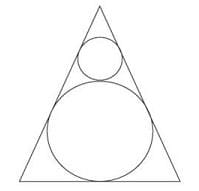A. π:36√3
B. π:18√3
C. π:27√3
D. π:42√3
Explanation.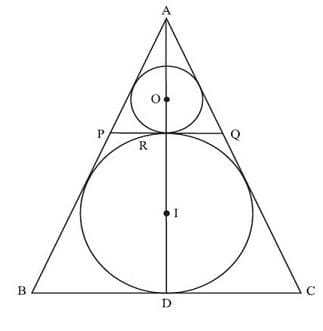In-radius of equilateral triangle of side a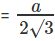Diameter of larger circle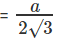Let us say common tangent PQ touches the two circle at R, center of smaller circle is I.
Now, PQ is parallel to BC. AR is perpendicular to PQ. Triangle PQR is also an equilateral triangle and AORID is a straight line. (Try to establish each of these observations. Just to maintain the rigour.)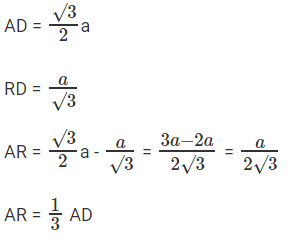Radius of smaller circle = 1/3 radius of larger circle
Radius of smaller circle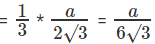Area of smaller circle = πr2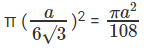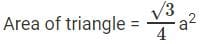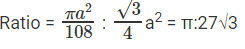The question is " What is the ratio of the area of the smaller circle to that of the equilateral triangle?"
Hence, the answer is π:27√3.
Choice C is the correct answer.

Question 5: Perimeter of a △ with integer sides is equal to 15. How many such triangles are possible?
A. 7
B. 6
C. 8
D. 5
Explanation.
Let us assume a ≤ b ≤ c.
a = 1, Possible triangle 1, 7, 7
a = 2, possible triangle 2, 6, 7
a = 3, possible triangles 3, 6, 6 and 3, 5, 7
a = 4, possible triangles 4, 4, 7 and 4, 5, 6
a = 5, possible triangle is 5, 5, 5
There are totally 7 triangles possible.
The question is "Perimeter of a △ with integer sides is equal to 15. How many such triangles are possible?"
Hence, the answer is 7.
Choice A is the correct answer.

Question 6There is an equilateral triangle with a square inscribed inside it. One of the sides of the square lies on a side of the equilateral △. What is the ratio of the area of the square to that of the equilateral triangle?
A. 12 : 12 + 7√3
B. 24 : 24 + 7√3
C. 18 : 12 + 15√3
D. 6 : 6 + 5√3
Explanation.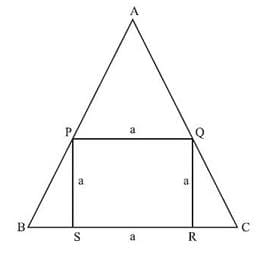APQ is an equilateral △. As PQ is parallel to BC.
Let side of the square be ‘a’
AP = a = AQ
△QRC has angles 30 – 60 – 90.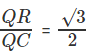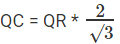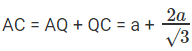Area of equilateral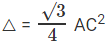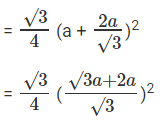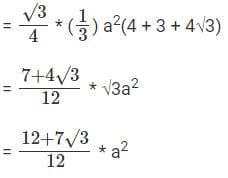Ratio of area of square to that of equilateral △ is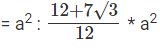= 12 : 12 + 7√3
The question is "What is the ratio of the area of the square to that of the equilateral triangle?"
Hence, the answer is 12 : 12 + 7√3.
Choice A is the correct answer.

Question 7: △ABC has integer sides x, y, z such that xz = 12. How many such triangles are possible?
A. 8
B. 6
C. 9
D. 12
Explanation.
xz = 12
x,z can be 1, 12 or 2, 6 or 3, 4
Possible triangles
1 - 12 - 12
2 - 6 - 5; 2 - 6 - 6; 2 - 6 - 7
3 - 4 - 2; 3 - 4 - 3; 3 - 4 - 4; 3 - 4 - 5; 3 - 4 - 6.
The question is "△ABC has integer sides x, y, z such that xz = 12. How many such triangles are possible?"
Hence, the answer is 9 triangles.
Choice C is the correct answer.

Question 8: ABCDE is a regular pentagon. O is a point inside the pentagon such that AOB is an equilateral triangle. What is ∠OEA?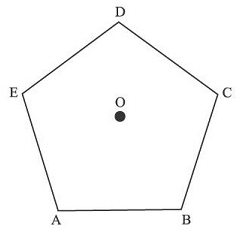A. 66°
B. 48°
C. 54°
D. 72°
Explanation.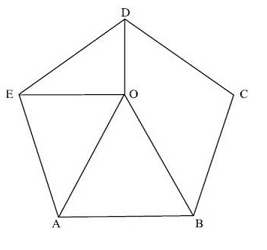Join OE and OD.
Internal angle of regular pentagon = 108°
∠EAB = ∠EDC = 108°
∠OAB = 60°
∠EAO = 48°
AO = OB = AB as the triangle is equilateral.
AB = AE as this is a regular pentagon.
Triangle AEO is isosceles as AO = EA.
∠AEO = ∠AOE = x (say)
In triangle AEO,
∠OAE + 2x = 180°
48° + 2x = 180°
2x = 132°, or x = 66°
The question is " What is ∠OEA?"
∠OEA = 66°
Hence, the answer is 66°
Choice A is the correct answer.

Question 9△ has sides a2, b2 and c2. Then the triangle with sides a, b, c has to be:
A. Right-angled
B. Acute-angled
C. Obtuse-angled
D. Can be any of these three
Explanation.

Assume a ≤ b ≤ c,
we have a2 + b2 > c2.
This implies the triangle with sides a, b, c has to be acute-angled.
The question is "△ has sides a2, b2 and c2. Then the triangle with sides a, b, c has to be:"
Hence, the answer is Acute-angled .
Choice B is the correct answer.

Question 10Consider a right–angled triangle with inradius 2 cm and circumradius of 7 cm. What is the area of the triangle?
A. 32 sq cms
B. 31.5 sq cms
C. 32.5 sq cms
D. 33 sq cms
Explanation.
r = 2
R = 7 (Half of hypotenuse)
Hypotenuse = 14
r = (a + b - h)/2
2 = (a + b - 14)/2
a + b - 14 = 4
a + b = 18
a2 + b2 = 14 2
a2 + (18 - a)2 = 14 2
a2 + 324 + a2 - 36a = 196
2a2 - 36a + 128 = 0
a2 - 18a + 64 = 0
Now the 2 roots to this equation will effectively be a, 18 – a. Product of the roots = 64.
Area = 1/2 * product of roots (How? Come on, you can figure this out.)
= 32 sq. cms
The question is "What is the area of the triangle?"
Hence, the answer is 32 sq. cms
Choice A is the correct answer.

The document Geometry Questions with Answer- 1 Notes | Study Quantitative Aptitude (Quant) - CAT is a part of the CAT Course Quantitative Aptitude (Quant).
All you need of CAT at this link: CAT

## Quantitative Aptitude (Quant)

163 videos|164 docs|131 tests
 Use Code STAYHOME200 and get INR 200 additional OFF

## Quantitative Aptitude (Quant)

163 videos|164 docs|131 tests

### How to Prepare for CAT

Read our guide to prepare for CAT which is created by Toppers & the best Teachers

Track your progress, build streaks, highlight & save important lessons and more!

,

,

,

,

,

,

,

,

,

,

,

,

,

,

,

,

,

,

,

,

,

;